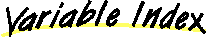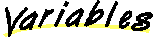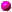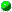Class com.oroinc.math.geometry.Tuple
`All Packages  Class Hierarchy  This Package  Previous  Next  Index`

# Class com.oroinc.math.geometry.Tuple

```java.lang.Object
|
+----com.oroinc.math.geometry.Tuple
```

public class Tuple
extends Object
implements Cloneable
A base class for representing points and vectors in homogeneous coordinates. Only tuples with dimensions between 1 and 4 are supported.

Copyright © 1996, 1997 Original Reusable Objects, Inc. All rights reserved.

Author:
Daniel F. Savarese

##_dimension__elements_

##Tuple(float, float)
Creates a two dimensional Tuple with the given coordinates.Tuple(float, float, float)
Creates a three dimensional Tuple with the given coordinates.Tuple(int)
Creates a Tuple of a given dimension and initizlies all of its coordinates to 0.Tuple(Tuple)
The Tuple copy constructor.

##_assign_(Tuple)
This is the assignment operator for Tuples.add(Tuple, Tuple)
Add two tuples and return the result.addTo(Tuple)
This method is the equivalent of the += operator.clone()
Returns a deep copy of the Tuple.dimension()
Returns the dimension of the Tuple.divide(float)
This method divides the Tuple by a scalar and returns a new Tuple containing the result.divideBy(float)
This method is the equivalent of the /= operator.element(int)
Serves the function of a read only [] operator.inverse()
This method is the equivalent of the unary - operator.multiply(float)
This method multiplies the Tuple by a scalar and returns a new Tuple containing the result.multiply(SquareMatrix)
This method multiplies the Tuple by a SquareMatrix and returns a new Tuple containing the result.multiplyBy(float)
This method is the equivalent of the *= operator.multiplyBy(SquareMatrix)
This method is the equivalent of the *= operator.setDimension(int)
Sets the dimension of the tuple.setElement(int, float)
Assigns a value to the Tuple element at the indicated index..subtract(Tuple, Tuple)
Subtract two tuples and return the result.subtractFrom(Tuple)
This method is the equivalent of the -= operator.toString()
Returns a string representation of the tuple including the homogeneous coordinate.zero()
Zeroes the elements of the tuple, preserving homogeneous coordinates.

##_elements_
```  protected float _elements_[]
```_dimension_
```  protected int _dimension_
```

##Tuple
```  public Tuple(Tuple tuple)
```
The Tuple copy constructor. Creates a new Tuple instance that is a deep copy of the argument.

Parameters:
tuple - The Tuple used to initialize the new Tuple.Tuple
```  public Tuple(int dimension)
```
Creates a Tuple of a given dimension and initizlies all of its coordinates to 0. Only dimensions 1 to 4 are supported and no check is made to ensure a valid argument was passed. It is the programmer's responsibility to use valid arguments.

Parameters:
dimension - The dimension of the new Tuple.Tuple
```  public Tuple(float x,
float y)
```
Creates a two dimensional Tuple with the given coordinates.

Parameters:
x - The first Tuple value.
y - The second Tuple value.Tuple
```  public Tuple(float x,
float y,
float z)
```
Creates a three dimensional Tuple with the given coordinates.

Parameters:
x - The first Tuple value.
y - The second Tuple value.
z - The third Tuple value.

##add
```  public final static Tuple add(Tuple tuple1,
Tuple tuple2)
```
Add two tuples and return the result.

Parameters:
tuple1 - The first addition operand.
tuple2 - The second addition operand.
Returns:
A new Tuple instance containing the sum of the two operands.subtract
```  public final static Tuple subtract(Tuple tuple1,
Tuple tuple2)
```
Subtract two tuples and return the result.

Parameters:
tuple1 - The first addition operand.
tuple2 - The second addition operand.
Returns:
A new Tuple instance containing tuple1 - tuple2._assign_
```  protected final void _assign_(Tuple tuple)
```
This is the assignment operator for Tuples. Assigns the value of the argument to the calling Tuple. It is the responsibility of the programmer to never assign a vector to a point or a point to a vector.

Parameters:
tuple - The value to assign to the Tuple.clone
```  public Object clone()
```
Returns a deep copy of the Tuple. Essentially just returns
```new Tuple(this);
```
Tuple implements the Cloneable interface principally as a convenience.

Returns:
A deep copy of the Tuple.
Overrides:
clone in class Objectdimension
```  public final int dimension()
```
Returns the dimension of the Tuple.

Returns:
The dimensions of the Tuple.element
```  public final float element(int index)
```
Serves the function of a read only [] operator. Returns the value of the appropriate Tuple element. No boundary checking is done, so the programmer must be sure to use a valid index.

Parameters:
The - index of the element to return.
Returns:
The element at the specified index of the Tuple.setElement
```  public void setElement(int index,
float scalar)
```
Assigns a value to the Tuple element at the indicated index..

Parameters:
index - The index of the element to change.
scalar - The new value to assign.setDimension
```  public void setDimension(int dimension)
```
Sets the dimension of the tuple. Only dimensions 1 to 4 are supported and no check is made to ensure a valid argument was passed. It is the programmer's responsibility to use valid arguments.

Parameters:
dimension - The dimension of the new Tuple.zero
```  public final void zero()
```
Zeroes the elements of the tuple, preserving homogeneous coordinates. In other words, if the object is a vector it becomes the zero vector, and if it is a point, it becomes the origin of the Cartesian coordinate system.addTo
```  public final void addTo(Tuple tuple)
```
This method is the equivalent of the += operator. It adds the argument to the calling Tuple.

Parameters:
tuple - The value to add to the Tuple.subtractFrom
```  public final void subtractFrom(Tuple tuple)
```
This method is the equivalent of the -= operator. It subtracts the argument from the calling Tuple.

Parameters:
tuple - The value to subtract from the Tuple.multiplyBy
```  public final void multiplyBy(float scalar)
```
This method is the equivalent of the *= operator. It multiplies the Tuple by the argument.

Parameters:
scalar - The value by which to multiply the Tuple.multiplyBy
```  public final void multiplyBy(SquareMatrix matrix)
```
This method is the equivalent of the *= operator. It multiplies the Tuple by the argument, a SquareMatrix.

Parameters:
matrix - The value by which to multiply the Tuple.divideBy
```  public final void divideBy(float scalar)
```
This method is the equivalent of the /= operator. It divides the Tuple by the argument.

Parameters:
scalar - The value by which to divide the Tuple.multiply
```  public final Tuple multiply(float scalar)
```
This method multiplies the Tuple by a scalar and returns a new Tuple containing the result.

Parameters:
scalar - The value by which to multiply the Tuple.
Returns:
The product of the Tuple and the scalar.multiply
```  public final Tuple multiply(SquareMatrix matrix)
```
This method multiplies the Tuple by a SquareMatrix and returns a new Tuple containing the result.

Parameters:
matrix - The value by which to multiply the Tuple.
Returns:
The product of the Tuple and the SquareMatrix.divide
```  public final Tuple divide(float scalar)
```
This method divides the Tuple by a scalar and returns a new Tuple containing the result.

Parameters:
scalar - The value by which to divide the Tuple.
Returns:
The result of the division of the Tuple by the scalar.inverse
```  public final Tuple inverse()
```
This method is the equivalent of the unary - operator. It returns the inverse (i.e., -1 times the argument) of the Tuple.

Returns:
The new Tuple equal to the inverse of the caller.toString
```  public final String toString()
```
Returns a string representation of the tuple including the homogeneous coordinate.

Returns:
A string representation of the tuple.
Overrides:
toString in class Object

`All Packages  Class Hierarchy  This Package  Previous  Next  Index`